# To graph : The figure provided below such that it is symmetric with respect to x -axis.### Precalculus: Mathematics for Calcu...

6th Edition
Stewart + 5 others
Publisher: Cengage Learning
ISBN: 9780840068071### Precalculus: Mathematics for Calcu...

6th Edition
Stewart + 5 others
Publisher: Cengage Learning
ISBN: 9780840068071

#### Solutions

Chapter 1.8, Problem 122E

(a)

To determine

## To graph: The figure provided below such that it is symmetric with respect to x -axis.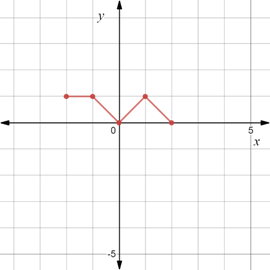Expert Solution

### Explanation of Solution

Given information:

The unsymmetrical graph is provided below,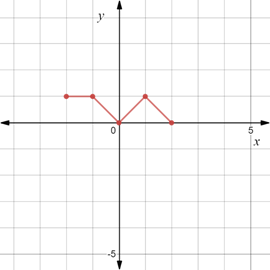Graph:

Reflect the provided graph about the x -axis such that the x -coordinate of each point remains the same and y -coordinate becomes negative.

The complete graph such that it is symmetric with respect to x -axis is provided below,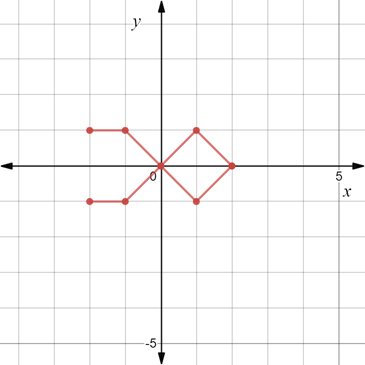Interpretation:

Graph of the equation is symmetric with respect to x -axis means that graph is not changed when reflected about the x- axis.

The graph of an equation is symmetric about the x -axis, when y is replaced by y , the graph of the equation remains unchanged.

(b)

To determine

Expert Solution

### Explanation of Solution

Given information:

The unsymmetrical graph is provided below,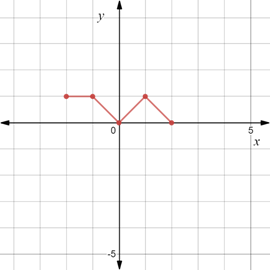Graph:

Reflect the provided graph about the y -axis such that the x -coordinate of each point becomes negative and y -coordinate remains the same.

The complete graph such that it is symmetric with respect to y -axis is provided below,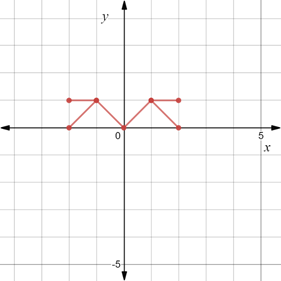Interpretation:

Graph of the equation is symmetric with respect to y -axis means that graph is not changed when reflected about the y- axis.

The graph of an equation is symmetric about the y -axis, when x is replaced by x , the graph of the equation remains unchanged.

(c)

To determine

Expert Solution

### Explanation of Solution

Given information:

The unsymmetrical graph is provided below,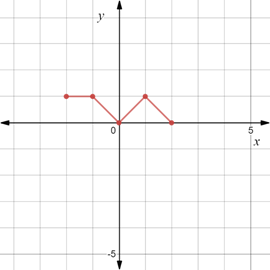Graph:

Reflect the provided graph about the origin such that the x -coordinate of each point becomes negative and y -coordinate also becomes negative.

The complete graph such that it is symmetric with respect to origin is provided below,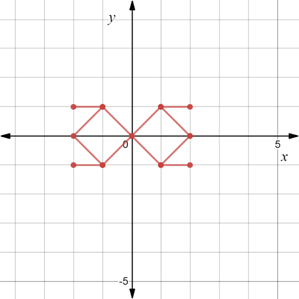Interpretation:

Graph of the equation is symmetric with respect to origin means that graph is not changed when rotated 180° about the origin.

The equation is symmetric with respect to origin, when y is replaced by y and x is replaced by x , the equation remains unchanged.

### Have a homework question?

Subscribe to bartleby learn! Ask subject matter experts 30 homework questions each month. Plus, you’ll have access to millions of step-by-step textbook answers!